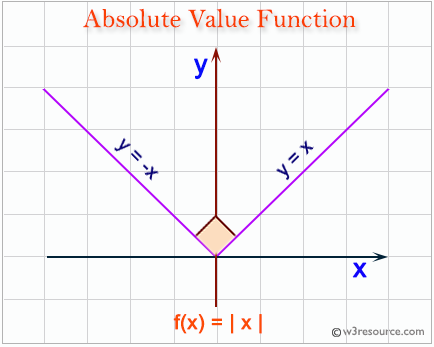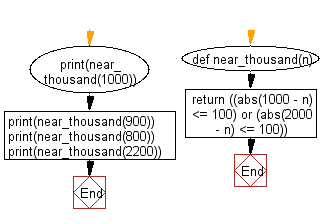﻿ Python: Test whether a number is within 100 of 1000 or 2000 - w3resource# Python: Test whether a number is within 100 of 1000 or 2000

## Python Basic: Exercise-17 with Solution

Write a Python program to test whether a number is within 100 of 1000 or 2000.

Python abs(x) function:

The function returns the absolute value of a number. The argument may be an integer or a floating point number. If the argument is a complex number, its magnitude is returned.Sample Solution:-

Python Code:

``````def near_thousand(n):
return ((abs(1000 - n) <= 100) or (abs(2000 - n) <= 100))
print(near_thousand(1000))
print(near_thousand(900))
print(near_thousand(800))
print(near_thousand(2200))
```
```

Sample Output:

```True
True
False
False ```

Flowchart:## Visualize Python code execution:

The following tool visualize what the computer is doing step-by-step as it executes the said program:

Python Code Editor:

Have another way to solve this solution? Contribute your code (and comments) through Disqus.

What is the difficulty level of this exercise?

Test your Python skills with w3resource's quiz

﻿

## Python: Tips of the Day

Try-catch-else construct:

```try:
foo()
except Exception:
print("Exception occured")
else:
print("Exception didnt occur")
finally:
print("Always gets here")
```